MATH 1280 Introduction to Statistics – Midterm Exam – part 2

Painted by Monet

Midterm Part 2 – questions 4 to 6 include their answers (total questions is 16)

Use this information for the next four questions: Researchers wanted to know about a standardized test that was used across the country. To get an estimate of the answer, they collected data from two classrooms of students who completed the test.
Scores from Classroom A:
8, 6, 9, 9, 8, 7, 8, 9, 10, 8, 10, 5, 4, 10, 9, 9

Scores from Classroom B:
9, 8, 7, 8, 9, 10, 9, 10, 7, 8, 10, 9, 9, 4, 6, 3

4) The set of all scores on the standardized test across the country is:
Select one:
a. a statistic
b. a parameter
c. the sample Incorrect
d. the population…> Correction

Monet’s painting

5) When all the scores for the two classrooms are added and divided by the number of observations, the resulting value is a:
Select one:
a. a statistic…> Correct
b. a parameter
c. the sample
d. the population

One of Monet’s paintings

6) What is the mean score in Group A?
Select one:
a. 8.4375
b. 8.3125
c. 8.0625…> Correct
d. 8.375
e. 8.4375 / sqrt(n)
R code:
> x <-c(8, 6, 9, 9, 8, 7, 8, 9, 10, 8, 10, 5, 4, 10, 9, 9)
> mean(x)
Result:
 8.0625

# To be continued to part 3

1.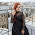Oh, I love Claude Monet, he's my favorite artist!
http://www.recklessdiary.ru

1.Thank you to visit:
MATH 1280 Introduction to Statistics – Midterm Exam – part 2
@
Painted by Monet

Midterm Part 2 – questions 4 to 6 include their answers (total questions is 16)

2.MATH 1280 Introduction to Statistics – Midterm Exam – part 2
-
6) What is the mean score in Group A?

Select one:
a. 8.4375
b. 8.3125
c. 8.0625…> Correct
d. 8.375
e. 8.4375 / sqrt(n)
R code:
> x <-c(8, 6, 9, 9, 8, 7, 8, 9, 10, 8, 10, 5, 4, 10, 9, 9)
> mean(x)
Result:
 8.0625

# To be continued to part 3

2.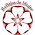Lovely Monet paintings!!!

1.Thank you to visit:
MATH 1280 Introduction to Statistics – Midterm Exam – part 2
@
Use this information for the next four questions: Researchers wanted to know about a standardized test that was used across the country. To get an estimate of the answer, they collected data from two classrooms of students who completed the test.
Scores from Classroom A:
8, 6, 9, 9, 8, 7, 8, 9, 10, 8, 10, 5, 4, 10, 9, 9

Scores from Classroom B:
9, 8, 7, 8, 9, 10, 9, 10, 7, 8, 10, 9, 9, 4, 6, 3

3.Monet's work is so beautiful and tranquil.

1.Thank you to visit:
MATH 1280 Introduction to Statistics – Midterm Exam – part 2
@
4) The set of all scores on the standardized test across the country is:
Select one:
a. a statistic
b. a parameter
c. the sample Incorrect
d. the population…> Correction

4.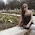Lovely paintings☺

1.Thank you to visit:
MATH 1280 Introduction to Statistics – Midterm Exam – part 2
@
5) When all the scores for the two classrooms are added and divided by the number of observations, the resulting value is a:

Select one:
a. a statistic…> Correct
b. a parameter
c. the sample
d. the population

5.these pictures are beautiful ☺
greetings
Lili

1.Thank you to visit:
MATH 1280 Introduction to Statistics – Midterm Exam – part 2
@
6) What is the mean score in Group A?
Select one:
a. 8.4375
b. 8.3125
c. 8.0625…> Correct
d. 8.375
e. 8.4375 / sqrt(n)
R code:
> x <-c(8, 6, 9, 9, 8, 7, 8, 9, 10, 8, 10, 5, 4, 10, 9, 9)
> mean(x)
Result:
 8.0625

# To be continued to part 3

6.Beautiful Monet paintings.
Kisses!

galerafashion.com

1.Thank you to visit:
MATH 1280 Introduction to Statistics – Midterm Exam – part 2
@
Painted by Monet

Midterm Part 2 – questions 4 to 6 include their answers (total questions is 16)

7.I've always admired those who understand mathematics.
It's all Greek to me.
Which is funny, because math isn't a language.
Or IS it?

1.Thank you to visit:
MATH 1280 Introduction to Statistics – Midterm Exam – part 2
@
Thank you to visit:
MATH 1280 Introduction to Statistics – Midterm Exam – part 2
@
Use this information for the next four questions: Researchers wanted to know about a standardized test that was used across the country. To get an estimate of the answer, they collected data from two classrooms of students who completed the test.
Scores from Classroom A:
8, 6, 9, 9, 8, 7, 8, 9, 10, 8, 10, 5, 4, 10, 9, 9

Scores from Classroom B:
9, 8, 7, 8, 9, 10, 9, 10, 7, 8, 10, 9, 9, 4, 6, 3

8.so cute paintings;)

1.Thank you to visit:
MATH 1280 Introduction to Statistics – Midterm Exam – part 2
@
4) The set of all scores on the standardized test across the country is:
Select one:
a. a statistic
b. a parameter
c. the sample Incorrect
d. the population…> Correction

9.Pretty paintings :)

1.Thank you to visit:
MATH 1280 Introduction to Statistics – Midterm Exam – part 2
@
5) When all the scores for the two classrooms are added and divided by the number of observations, the resulting value is a:

Select one:
a. a statistic…> Correct
b. a parameter
c. the sample
d. the population

10.Ciekawy test :)

1.Thank you to visit:
MATH 1280 Introduction to Statistics – Midterm Exam – part 2
@
6) What is the mean score in Group A?
Select one:
a. 8.4375
b. 8.3125
c. 8.0625…> Correct
d. 8.375
e. 8.4375 / sqrt(n)
R code:
> x <-c(8, 6, 9, 9, 8, 7, 8, 9, 10, 8, 10, 5, 4, 10, 9, 9)
> mean(x)
Result:
 8.0625

# To be continued to part 3

11.Belas pinturas e aproveito para desejar um bom Domingo.

Andarilhar
Dedais de Francisco e Idalisa

1.Thank you to visit:
MATH 1280 Introduction to Statistics – Midterm Exam – part 2
@
Painted by Monet

Midterm Part 2 – questions 4 to 6 include their answers (total questions is 16)

12.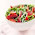Thanks for sharing this interesting information.

1.Thank you to visit:
MATH 1280 Introduction to Statistics – Midterm Exam – part 2
@
Use this information for the next four questions: Researchers wanted to know about a standardized test that was used across the country. To get an estimate of the answer, they collected data from two classrooms of students who completed the test.
Scores from Classroom A:
8, 6, 9, 9, 8, 7, 8, 9, 10, 8, 10, 5, 4, 10, 9, 9

Scores from Classroom B:
9, 8, 7, 8, 9, 10, 9, 10, 7, 8, 10, 9, 9, 4, 6, 3

2.MATH 1280 Introduction to Statistics – Midterm Exam – part 2

13.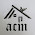Gostei das pinturas.
Boa continuação de semana.

1.Thank you to visit:
MATH 1280 Introduction to Statistics – Midterm Exam – part 2
-
4) The set of all scores on the standardized test across the country is:
Select one:
a. a statistic
b. a parameter
c. the sample Incorrect
d. the population…> Correction

14.Sounds interesting data collection to get to results.

1.Thank you to visit:
MATH 1280 Introduction to Statistics – Midterm Exam – part 2
-
5) When all the scores for the two classrooms are added and divided by the number of observations, the resulting value is a:
Select one:
a. a statistic…> Correct
b. a parameter
c. the sample
d. the population